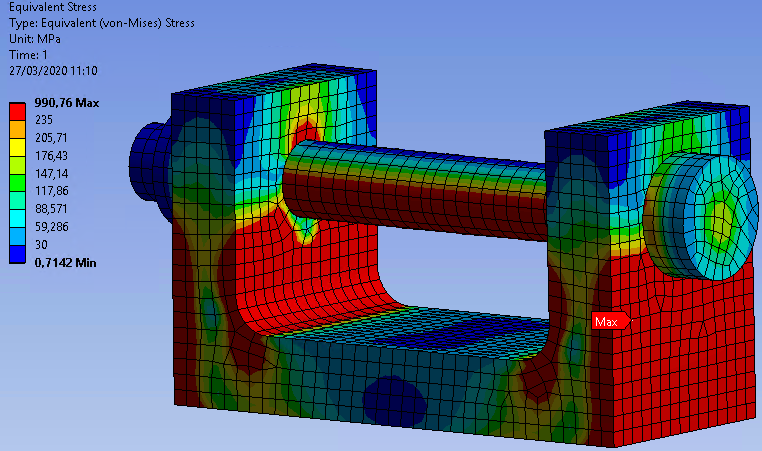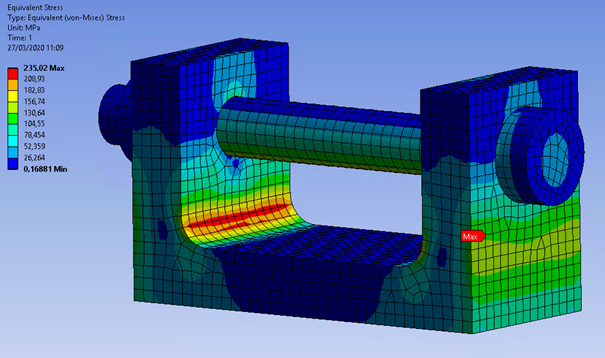• 2.6K Views
• Last Post 27 March 2020
• Topic Is Solved
Rashi posted this 31 July 2019

Hi,

I'm simulating the effect of bolt pre-tension load on a geometry which consists of a nut and a bolt. I have covered the standard bolt torque to bolt the pretensions force using an equation. Afterward, I got to know its more accurate to apply the torque using a "stretch control" concept. Where the stretch of the bolt section is measured.

In ANSYS when applying the bolt pretension it can be applied as "adjustment". So what I want to know is, 1) does this adjustment equal to the actual bolt stress when the bolt is under pretension force?

2) Also, how does ANSYS calculate adjustment value to a given bolt pretension force?

Thank you.

peteroznewman posted this 31 July 2019

If you have two faces on the bolt shank, one between the head and the nut, and a second face that is in the nut, then you can select the face above the nut and apply a Bolt Pretension.

In Workbench, you can enter a Pretension force in the bolt shank. It will automatically split the face, put a node on each side and pull them together to achieve the requested force.

This is usually done in step 1 of a two step analysis. In step 2, the bolt pretension is changed from Pretension Force to Lock. That holds the automatically calculated adjustment value fixed while other loads can be applied to the structure in step 2.

Rashi posted this 01 August 2019

So does this mean what shown in the below picture?I have applied 10000N to the following bolt shank and got the following adjustment value 0.11129 mm. Next, I checked the displacement in the axial direction of the bolt (0.10625 mm), but that value does not equal to the adjustment value (0.11129 mm).

Or does it to be equal to the total displacement?

0.10625 mm + MAG(-5.72E-3 mm) = 0.11197 mm

Thank you.

Best Regards,

Rashi

Attached Files

Rashi posted this 05 August 2019

Mr. Peter, I have attached the original image for better viewing.

Attached Files

peteroznewman posted this 05 August 2019Rashi posted this 09 August 2019

Mr. Peter,

I did a simulation as you have explained. But I still cannot figure out how the adjustment is calculated. Because when I add the bolt stretch I get wrong results.

I have also attached the project file below.

Thank you

Attached Files

peteroznewman posted this 10 August 2019

The problem you have created wants to bend the fastener.

You have added boundary conditions that artificially prevent the bending.

I don't like this configuration for understanding something simple like bolt adjustment.

I recommend you use a block with a hole in it. Tighten the bolt on the block, then you don't need artificial boundary conditions.

Rashi posted this 10 August 2019

Mr. Peter,

How do you calculate the "bolt stretch" which you have mentioned above?

I have corrected the simulation and attached it below.

Thank you

Attached Files

peteroznewman posted this 11 August 2019

• Take a shaft, 9.6 mm diameter, 200 mm long.
• Put a Fixed Support at both ends of the shaft.
• Apply a bolt pretension of 25000 N.
• Edit the Material to have Poisson's Ratio = 0 so the cross-sectional area doesn't change under load.An almost exact match!Rashi posted this 11 August 2019

Mr. Peter,

Thank you for the detailed explanation.

I now understand how adjustment is calculated.

How ever if the adjustment is calculated like this(only considering the deformation of the bolt without considering the deformation of the clamped object) , is this the reason why there is larger discrepancy between the adjustment value and real displacement when there is clamped object? If so, in a real world case if we use the adjustment value to apply the bolt force we would have a higher force than the rated force right?

peteroznewman posted this 11 August 2019

In the example I described above, there is no clamped object.

This was created as the simplest example of adjustment from an applied Bolt Preload Force.

Adjustment is the contraction required at the center of bolt to achieve the pretension force entered.

Change the example above and let just one end have a Fixed Support and the other end have a spring to ground, with a 25000 N/mm stiffness. The spring represents the clamped object. Now the adjustment is 1 mm to compress the spring to 25000 N plus the 0.3457 mm to stretch the bolt.If you apply an Adjustment instead of a Preload Force, you can find out the force in the bolt by plotting the Working Load.

Please mark one of the posts with Is Solution to show this discussion as Solved, or ask a followup question.

Rashi posted this 11 August 2019

Mr. Peter,

Thank you for your detailed explanation.

Best Regards,

Rashiga

trungnghiale94 posted this 25 March 2020

Mr. Peter,

I have a problem when calculate the preadjustment from the preLoad using your formula. Then, the stress value when I use the preload is way greater than when I use the preadjustment (8 times ). So I would like to know which one is more reliable ? because it seems to me that every time I use the preload calculated from the torque, I always obtain locally a significant value of the stress

peteroznewman posted this 25 March 2020

"every time I use the preload calculated from the torque, I always obtain locally a significant value of the stress"

What formula do you use to calculate preload from torque?

I don't mention torque at all in the above discussion.

trungnghiale94 posted this 27 March 2020

Mr Peter,

• For your question, i used the relationship between the bolt pretension and torque. If not, there are tables like this one below available (  I typically obtain the same value with these two methods, so for me those are relatively reliable ). I used it because in practice, i often need to convert the torque value to the pretension one for the simulation.trungnghiale94 posted this 27 March 2020

•   In fact, what I really wanted to mention here is the difference between stress values of the two approaches ( pretension vs preadjustment) using your formula. For example,I do a simple test with :

- a shaft, 10 mm diameter                      - Bolt length : 70 mm ( btw, the length here must be just the face above the nut, isn't it ? )                      - Bolt pretension : 25000 N                      - Material : same as yours (E = 2.10e11 pa, v= 0 )                      -Contact between nut and bolt shank : bonded, the rest : Frictional 0.15 coef fric) => preadjustment : 0,11 mm   So here is what i obtain : PRETENSION 25000 N :PREADJUSTMENT : 0,11 mmSo that's why I wonder which method is more reliable ?  because when using pretension, I always obtain a significant stress value.  Thank in advance for your answer

peteroznewman posted this 27 March 2020

Please delete the duplicate posts and leave just one.

Your model is very different from my first example.  In my first example, there is only a shaft and nothing else.  The ends of the shaft are Fixed.  The point of that was to show the relationship between the requested preload and the resultant adjustment when the clamped part was infinitely stiff and did not deform at all because it was modeled as two fixed supports.

Your model is very different from my second example.  In my second example, a spring represents the clamped part. You have a U shaped part as the clamped part. The point of my second example was to show the relationship between the deformation of the part, modeled as a spring, under the preload and to add that to the stretch of the bolt then do a hand calculation on the Adjustment.

Your model has the peak stress in the base of the two arms that rise up from the base.  If you double the thickness of the two arms, the stress would go down a lot.  If you fill in the center of the U-shaped part so it is a solid block with a hole in it, the stress would go down a lot more.

I have not explained my second example sufficiently for you to understand that Adjustment is the sum of two deformations: Bolt Stretch and Part Compression. These two components each have their own stiffness values: Kbolt and Kpart.  These two springs are in series. The equivalent spring rate for springs in series is 1/Keq = 1/Kbolt + 1/Kpart.   My first example shows how to calculate Kbolt (shown as k in that example).  In my second example, I created the part by using a spring and was able to type in Kpart.  In your model, you would have to divide the Pretension force, F = 25kN, by the change in the distance between the holes on the outside of the U-shaped part to calculate Kpart to use with Kbolt to calculate Keq.

Then you will find that the Adjustment = F/Keq

trungnghiale94 posted this 27 March 2020

"Adjustment is the sum of two deformations: Bolt Stretch and Part Compression" - that says everything.

Thank you for your explanation. I understand now.

So I imagine that if we do the simulation with bolt pretension in assemblies including different elements, il will be very more complex and take a lot of time to calculate the Keq, so the best solution here is to use the Pretension instead of preadjustment most of the time, isn't it ?

peteroznewman posted this 27 March 2020

There is not much difference in computation time for setting Pretension Force vs using Adjustment.  The default is Preload Force because that is what is created when a Bolt Torque is specified on a drawing. The Adjustment is generally unknown because no one bothers to compute Kpart and Kbolt to compute Keq to use the Preload Force that is calculated from the Bolt Torque in order to input an Adjustment.  Just input the Preload Force.

trungnghiale94 posted this 27 March 2020

Yes. I meant it take time to calculate the Keq ( not the computation time )

Anyway, it 's clear to me now.